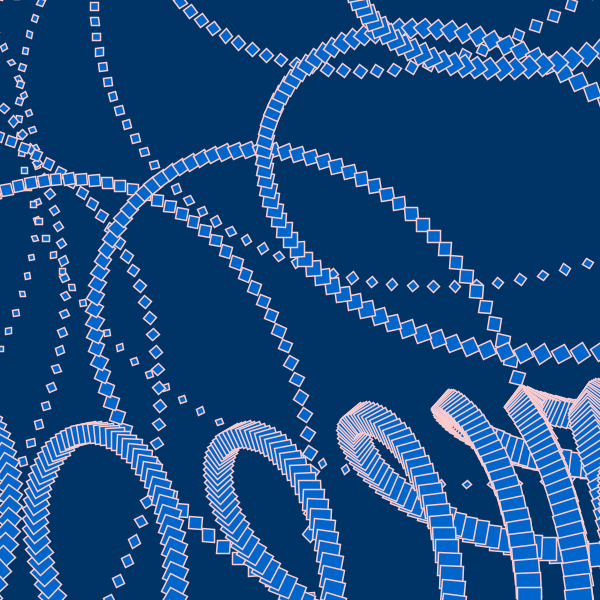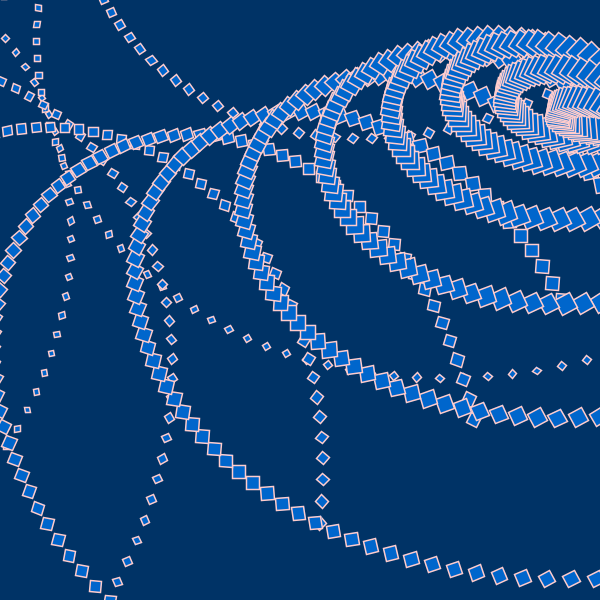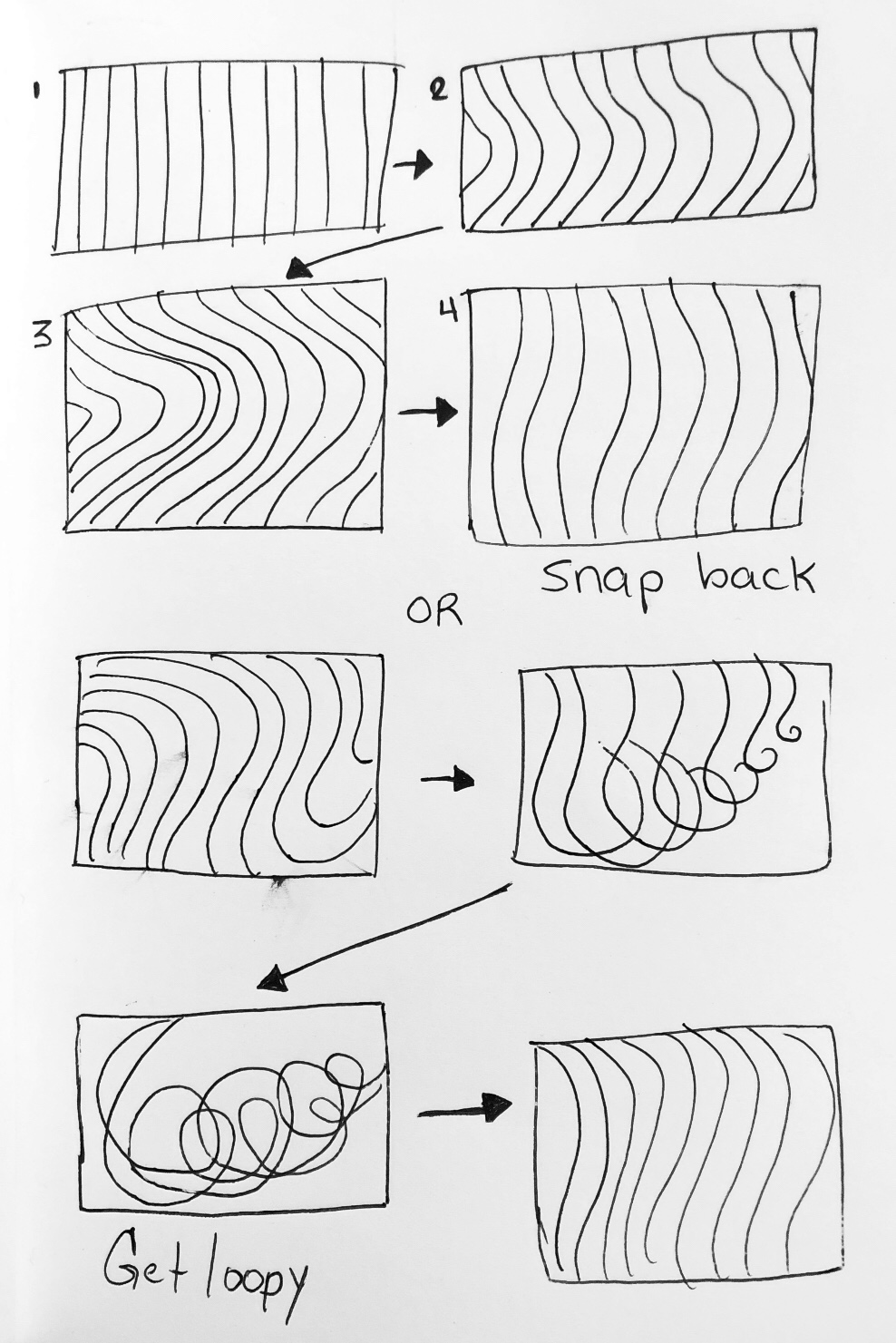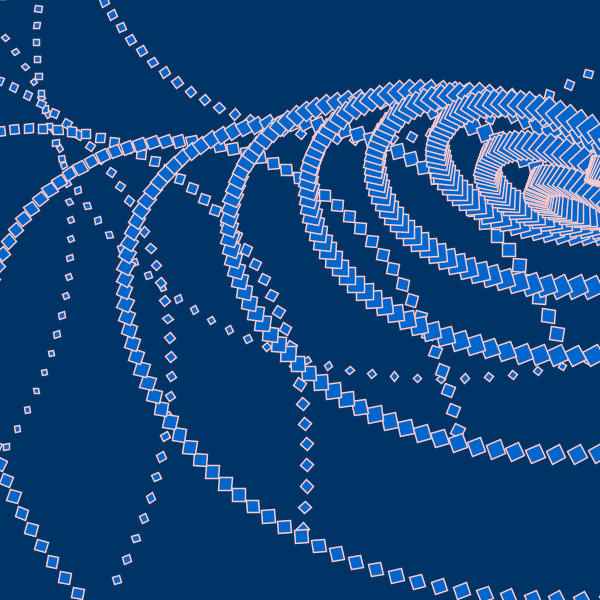# zaport-AnimatedLoopThis animated loop began as an undulating sine wave and took many different forms in the process of getting to where it is now. When I sketched out the loop I was intending for the lines to have an elastic quality, so that they would look as if they were being pulled in one direction and then let go. As I began to experiment with different arrangements, I found the rotate function to be interesting. What I ended up with were two rotated sine waves. The easing function that I selected was the PennerEaseInBack. It elastic-quality to the easing, which makes me like to think of the form as a slinky. This loop is completely abstract and combines 2D shapes to create a larger shape that exists in space.

Here are my sketches:This piece succeeds in its elastic-like movement and ability to create a sense of space. The easing function is a very helpful tool in creating these effects. However, it falls short in a number of ways. From a design perspective, the frame is cluttered and the motion is not so apparent. From a technical perspective, the code is not very sophisticated. I spent a majority of my time trying to figure out how the framework functions and how Processing, generally speaking, works.

Here’s the animated GIF:Here’s the code:

```  // This is a template for creating a looping animation in Processing/Java. // Zaport Week 2 60-212 //Template from Golan Levin //Thank you to Aaron Davey, Peter Sheehan, and Ty for theor help with this project   //When you press the 'F' key, this program will export a series of images // into a "frames" directory located in its sketch folder. // These can then be combined into an animated gif. // Known to work with Processing 3.3.6 // Prof. Golan Levin, January 2018   //=================================================== // Global variables. String myNickname = "nickname"; int nFramesInLoop = 120; int nElapsedFrames; boolean bRecording;   //=================================================== void setup() { size (600, 600); bRecording = false; nElapsedFrames = 0; }   //Credit Golan Levin float function_PennerEaseInBack (float q) {   float s = 1.70158; float d = q*q*((s+1.0)*q - s); return d; }   //=================================================== void keyPressed() { if ((key == 'f') || (key == 'F')) { bRecording = true; nElapsedFrames = 0; } }   //=================================================== void draw() {   // Compute a percentage (0...1) representing where we are in the loop. float percentCompleteFraction = 0; if (bRecording) { percentCompleteFraction = (float) nElapsedFrames / (float)nFramesInLoop; } else { percentCompleteFraction = (float) (frameCount % nFramesInLoop) / (float)nFramesInLoop; }   // Render the design, based on that percentage. renderMyDesign (percentCompleteFraction);   // If we're recording the output, save the frame to a file. if (bRecording) { saveFrame("frames/" + myNickname + "_frame_" + nf(nElapsedFrames, 4) + ".png"); nElapsedFrames++; if (nElapsedFrames &gt;= nFramesInLoop) { bRecording = false; } } }   //=================================================== void renderMyDesign (float percent) { // // YOUR ART GOES HERE. // This is an example of a function that renders a temporally looping design. // It takes a "percent", between 0 and 1, indicating where we are in the loop. // This example uses two different graphical techniques. // Use or delete whatever you prefer from this example. // Remember to SKETCH FIRST!   //---------------------- // here, I set the background and some other graphical properties background (0,51,102); smooth(); stroke (255,204,204); fill (0,102,204); strokeWeight (1.5);   //---------------------- // Here, I assign some handy variables. float cx = width/2; float cy = height/2; float r = 5; float m = millis(); float x = cx+r*cos(m); float y = cy+r*sin(m); float highlight = 0; float pos = 0;   //----------------------   // Slinky sin wave for (int sy=0; sy &lt;= height+2000; sy+=18) { float t = map (sy, 0, height, 0.0, 0.60); for (float x1= -2000 + 50.0 * cos ((t + percent)*TWO_PI); x1 &lt;= width; x1+=18) {   rotate(function_PennerEaseInBack (.02)); rect (x1, sy, r, r); rotate(.029); } r = r+.6; } } //=================================================== // Taken from https://github.com/golanlevin/Pattern_Master float function_DoubleExponentialSigmoid (float x, float a) { // functionName = "Double-Exponential Sigmoid";   float min_param_a = 0.0 + EPSILON; float max_param_a = 1.0 - EPSILON; a = constrain(a, min_param_a, max_param_a); a = 1-a;   float y = 0; if (x&lt;=0.5) { y = (pow(2.0*x, 1.0/a))/2.0; } else { y = 1.0 - (pow(2.0*(1.0-x), 1.0/a))/2.0; } return y; }```